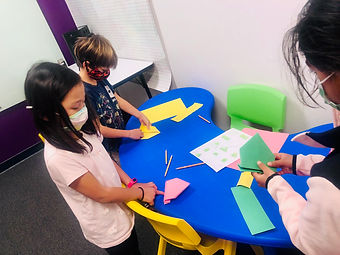## Ms. AJ

### Target 1​

###### Lesson Type:

Continuation

Number Operation

:

Computation

Solve multi-step problems within 100 (addition, subtraction, multiplication, and division).

###### 1:

Solve problems that have more than one operation.

###### 2:

Understand that the presence of parenthesis in an arithmetic equation signals for this part of the problem to be solved first.

###### 3:

Understand when solving a problem that has both addition and subtraction operations that solving starts on the left and continues to the right.

5th

###### Vocabulary:

PEMDAS, Order of Operations, Exponents, Powers of Two (Squares)

Activities:

Students continued working with PEMDAS (order of operations/parenthesis, exponents, multiplication, division, addition, subtraction) and practiced problems in which they had to break problems down and figure out what parts to solve first.

Students understood that multiplication and division are on the “same level” and what determines which goes first between them is which comes first reading the problem left to right. This is also true of addition and subtraction.

Students are introduced to exponents, learning about “powers of 2” or “squaring” numbers.

Students created their own order of operation equations to solve, then had other students solve theirs.### Home Exploration

###### Guiding Questions:## Absent Students:

### Target 2

:

###### 1:

Understand that multiplying numbers makes numbers larger.

###### 2:

Understand that multiplication represents the combination of equal groups of objects.

###### 3:

Understand that the same quantity can be group in multiple ways, creating different multiplication equations.

###### 4:

Interpret products of whole numbers as the total number of objects arranged into equal groupings.

###### 5:

Understand that the same process is utilized to multiply 3 or more whole numbers that is used to multiply only two numbers (i.e., 2x3x4 = 2 groups of 3 in 6, and 4 groups of 6 is 24).

4th

###### Vocabulary:

Activities:

Practiced multiplying numbers by playing "Multiplication War", a version of the game "War" in which the higher product between a pair of cards drawn by each player wins the round.

Students learned how to use the "magic square" method in solving multiplication problems involving two-digit numbers.### Home Exploration

###### Guiding Questions:### Target 3

:

###### 1:

Think about objects in 3D and draw conclusions about them from limited information.

###### 2:

Imagine an object being manipulated in space (rotated, folded, cut, etc.)

###### 3:

Use spatial reasoning to solve problems.

###### 4:

Form a mental image.

###### 5:

Transform mental images in some way.

3rd

###### Vocabulary:

perspective, compare

Activities:

Students followed step-by-step diagrams in order to create 3D origami frogs out of construction paper.### Home Exploration